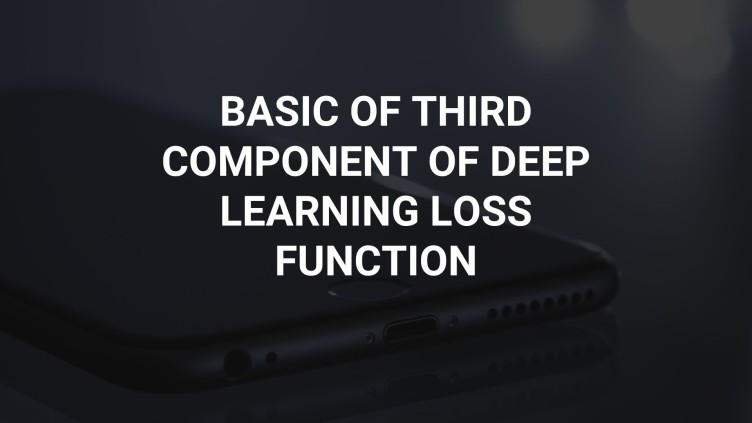## BASIC OF THIRD COMPONENT OF DEEP LEARNING LOSS FUNCTION

In the previous post we have discussed that we need to figure out three main component to design and develop deep learning models which are dataarchitecture, and loss function.After figuring out all three components calculation will be handle by framework which you are using and it will give you your desired model.We already write post's on data and architecture now we only need to figure out loss function.

What is Loss Function?

Loss is a criteria to judge our model performance that's by some framework also called it criterion function.It give us value and shows us how much far our model is from actual values.It gives a sense of our model efficiency and performances.Technically speaking it is just a mathematical function which take our model output and actual labeled value and compute difference to give as values.This mathematical function highly depends on label data types means choice of this mathematical functions depends on label data.Let say if we have a label which continuous like we want to predict sales price than it is good to use RMSE(Root Mean Square Error) and if you want to classify between different classes you have classes label.Let say in MNIST we have 10 classes in which we want to distinguished our input data then we will use categorical-entropy loss function.Main aim of loss function is to improve our model from its current state.

We can also define metrics to calculate our loss value in which a human can understand.Let say you have loss function for categorical loss(loss for classes) which gives you loss function as 7:7,then what does you interpret? To make your understanding easy we define metrics.Here in this case we use accuracy,which shows how accurate our model is.This accuracy is calculated from loss function only.In MNIST data example it will now show 94.5% accurate which gives us deeper understanding.

Differentiation of loss function?

Loss function's main aim is to minimizes error rate in deep learning model.which is achieved through differentiation and after which optimization.Differentiation is a mathematical process to calculate rate of change of something with respect to something.As we are taking differentiation on loss function which actually calculate how much our model is good.By taking differentiation on loss function we actually see that by changing little amount of our weights and bias how much our model is improved and in which direction we need to change our weights and bias to increase more our model efficiency.By seeing direction we change weights and biases through optimization program(OPTIMIZER).

Perceptual understanding of Differentiation in deep learning?

Let say your friend is lost in a jungle and he is trapped somewhere in jungle and continously calling you from there, you heard his voice slowly and you follow his voice and go in that direction and finally taking steps towards that direction you finally reached to your friends and you are very happy.Likewise in deep learning your model accuracy and efficiency is somewhere lost in the jungle and differentiation is a voice of your models efficiency which gives you direction to reach to your model efficiency and finally taking steps towards it will take you to model efficiency.Taking steps is the part of optimizer.

Popular loss functions are:-

RMSE:-Root Mean Square Error is mathematical function which first takes squares of prediction minus actual then take mean of it and then take square root of it.Its formula isRMSE=(scientific equation in book).

Here y is prediction

yi is actuals

n is number of samples.

It is mainly used in Linear Regression problem to find out continuous number.

binary_crossentropy/categorial_crossentropy:-This loss function is mainly used to classify input to a set of predefined classes.Its formula isLog Loss =(scintific equation in book)

It is sometimes also called log loss when yi is defined as actuals and y is represented as predictions.

Custom loss:-You can also defined custom loss which is basically the combination of above two losses or you can create your own loss also.

Conclusion:-In this post we have discussed and defined Loss function in details.We have also defined metrics and some of the loss function in details like RMSE loss and log loss functions.We have also saw the role of differentiation in deep learning models.